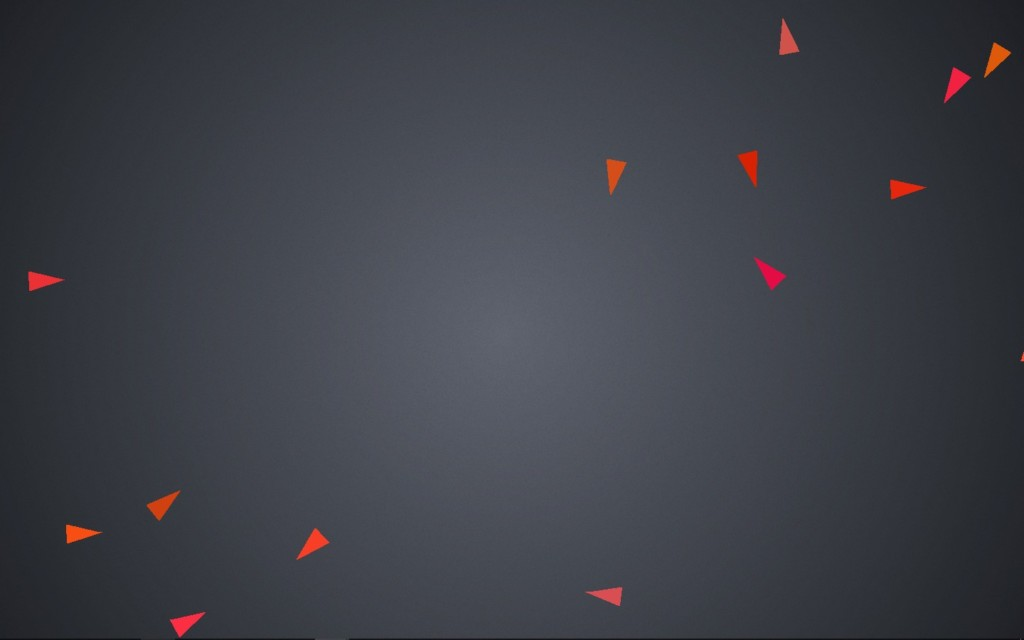After I finished my last exam and went home for the break, I was sitting at home in front of my fireplace screensaver in my room, certainly enjoying the freedom, yet knowing something was missing. Suddenly, it hit me. Christmas is a time to be together and enjoy one another’s company, not to hide away from others! So, I started working right away on a computer program to simulate “friends.”

This is the result:

## What is going on?

When the program is run, a number of creatures are spawned on the screen with random coordinates and orientation. Then, they abide by a number of rules that dictate how they move.

• Rule 1: Each creature likes to move in the direction that it is facing, represented by the pointy end of the triangles.
• Rule 2: The creatures like to be close to one another, but not too close.
• Rule 3: The creatures like to face the same direction as the creatures around them.
• Rule 4: If the creatures pass the edge of the screen, they will wrap over to the other side.

I didn’t specify that the creatures tend to move in flock-like formations, or that they tend to break apart at the edges of the screen. And yet, even with random placement and orientation, we can see that the creatures fall into the same patterns consistently.

The program I made can be categorized as an example of emergent behavior. By specifying rules for individual parts of a system and observing how that system behaves as a whole, we are able to study emergent behavior.

## A Full Explanation for Nerds

But how can we simulate such complex behavior? This program would essentially need four steps:

• Step 1: Define what a creature is, spawn some number of them into the level randomly.
• Step 2: Determine which rules apply to every creature.
• Step 3: Enforce rules.
• Step 4: Repeat steps 2 and 3.

One way to approach Step 1 is to create a data structure with everything a creature needs to exist. For movement, this includes force, velocity, and position. Each creature also has a color to make it distinguishable from its neighbors.

``````struct creature {
site force;
site velocity;
site position;
float angle;
colo color;
};
``````

For easy access, I made another data structure for containing every creature; every creature is numbered from zero to at most 500.

``````struct creature_set {
creature ref;
};
``````

Spawning them is very simple. I used a loop to fill a creature_set with 150 creatures, creating random values for position, angle, and color for each. The `%` operator is the remainder function; dividing a random integer from 0 to 32767 by 1440 will leave a pseudo-random remainder between 0 and 1440.

``````for (int i = 0; i < 150; i++) {
//randomize position
MyCreatureSet.ref[i].position.x = rand() % 1440;
MyCreatureSet.ref[i].position.y = rand() % 900;
//randomize angle
float r = static_cast <float> (rand()) / static_cast <float> (RAND_MAX);
MyCreatureSet.ref[i][i].angle = r * (2 * pi); //in radians
//randomize color
MyCreatureSet.ref[i].color.r = 205 + rand() % 50;
MyCreatureSet.ref[i].color.g = 0 + rand() % 90;
MyCreatureSet.ref[i].color.b = 0 + rand() % 90;
}``````

This gives us something like this:Next, the program needs to be able to go through each creature and enforce the rules. Rules 2 and 3, in particular, needed a clever solution. These rules make the creatures communal, and thus need the creatures to interact with each other. To do this, for each creature the program checks its distance from every other creature. An average position and angle are calculated from every other creature within 400 pixels.

Then, with a bit of trigonometry, the magnitude and direction of the Rule 2 force is calculated based off of a creature’s distance from the flock center. The expression `(Distance_From_Flock_Center) / -200) + 0.2)`makes the magnitude of the force positive (e.g. push from the center) if the creature is very close to the flock center, but negative (e.g. pull to center) otherwise.

```for (int i = 0; i < 150; i++) {
for (int j = 0; j < 150; j++) {
if (i != j) {
flock_center_x += MyCreatureSet.ref[j].position.x;
flock_center_y += MyCreatureSet.ref[j].position.y;
average_flock_angle += MyCreatureSet.ref[j].angle;
flock_count++;
}
}
}
if (flock_count > 0) {
flock_center_x /= flock_count;
flock_center_y /= flock_count;
site flock_center; flock_center.x = flock_center_x; flock_center.y = flock_center_y;
magnitude = ((GetDistance(flock_center, MyCreatureSet.ref[i].position) / -500) + 0.3) * 0.1;
direction = atan2(MyCreatureSet.ref[i].position.y - flock_center_y, MyCreatureSet.ref[i].position.x - flock_center_x);
average_flock_angle /= flock_count;
}
}```

The creature is turned towards the flock’s average angle (Rule 3), and the net force on a creature is calculated as a combination of its forward movement (Rule 1) and flocking movement (Rule 2).

Finally, the program adds the net force acting on it to its current velocity, which it adds to the position. This is a concept from physics that will move the objects according to Newton’s laws.

If you are interested in emergent behavior or the source code, feel free to talk to me or send an email! My email is 19046@jcpstudents.org.